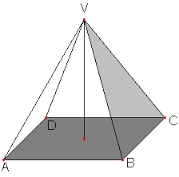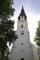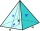# The bus stop

The bus stop waiting room has the shape of a regular quadrilateral pyramid 4 m high with a 5 m base edge. Calculate how much m2 roofing is required to cover the sheathing three walls, taking 40% of the additional coverage.

S =  49.5284 m2

### Step-by-step explanation:Did you find an error or inaccuracy? Feel free to write us. Thank you!Tips to related online calculators
Pythagorean theorem is the base for the right triangle calculator.

#### You need to know the following knowledge to solve this word math problem:

We encourage you to watch this tutorial video on this math problem:

## Related math problems and questions:How many square meters are needed to cover the shape of a regular quadrangular pyramid base edge 10 meters if the deviation lateral edges from the base plane are 68°? Calculate waste 10%.
• The roofThe roof of the tower has the shape of a regular quadrangular pyramid, the base edge of which is 11 m long and the side wall of the animal with the base an angle of 57°. Calculate how much roofing we need to cover the entire roof, if we count on 15% waste
• The tentThe tent shape of a regular quadrilateral pyramid has a base edge length a = 2 m and a height v = 1.8 m. How many m2 of cloth we need to make the tent if we have to add 7% of the seams? How many m3 of air will be in the tent?
• The tentCalculate how much cover (without a floor) is used to make a tent that has the shape of a regular square pyramid. The edge of the base is 3 m long and the height of the tent is 2 m.
• Church roofThe roof of the church tower has the shape of a regular tetrahedral pyramid with base edge length 5.4 meters and a height 5 m. It was found that needs to be corrected 27% covering of the roof area. What amount of material will be required?
• TowerThe top of the tower is a regular hexagonal pyramid with base edge 8 meters long and a height 5 meters. How many m2 of the sheet is required to cover the top of the tower if we count 8% of the sheet waste?
• Roof 7The roof has the shape of a regular quadrangular pyramid with a base edge of 12 m and a height of 4 m. How many percent is folds and waste if in construction was consumed 181.4m2 of plate?
• The plaster castThe plaster cast has the shape of a regular quadrilateral pyramid. The cover consists of four equilateral triangles with a 5 m side. Calculate its volume and surface area.Find the volume and surface of a regular quadrilateral pyramid if the bottom edge is 45 cm long and the pyramid height is 7 cm.
• Tetrahedral pyramidWhat is the surface of a regular tetrahedral (four-sided) pyramid if the base edge a=16 and height v=16?We have a regular quadrilateral pyramid with a base edge a = 10 cm and a height v = 7 cm. Calculate 1/base content 2/casing content 3/pyramid surface 4/volume of the pyramidCalculate the volume and surface area of a regular hexagonal pyramid with a base edge a = 30 m and a side edge b = 50 m.2/4 of the area of ​​the roof-shaped regular tetrahedral pyramid with base edge 10 m and height of 4 m is already covered with roofing. How many square meters still need to be covered?A regular quadrilateral pyramid has a volume of 24 dm3 and a base edge a = 4 dm. Calculate: a/height of the pyramid b/sidewall height c/surface of the pyramidHow many m2 of roofing is needed to cover a cone-shaped roof with a diameter of 10 m and a height of 4 m? Add an extra 4% to the overlays.Find the height and surface of a regular quadrilateral pyramid with a base edge a = 8cm and a wall height w = 10cm. Sketch a picture.Above the pavilion with a square ground plan with a side length of a = 12 m is a pyramid-shaped roof with a height v = 4.5 m. Calculate how much m2 of sheet metal is needed to cover this roof if 5.5% of the sheet we must add for joints and waste.